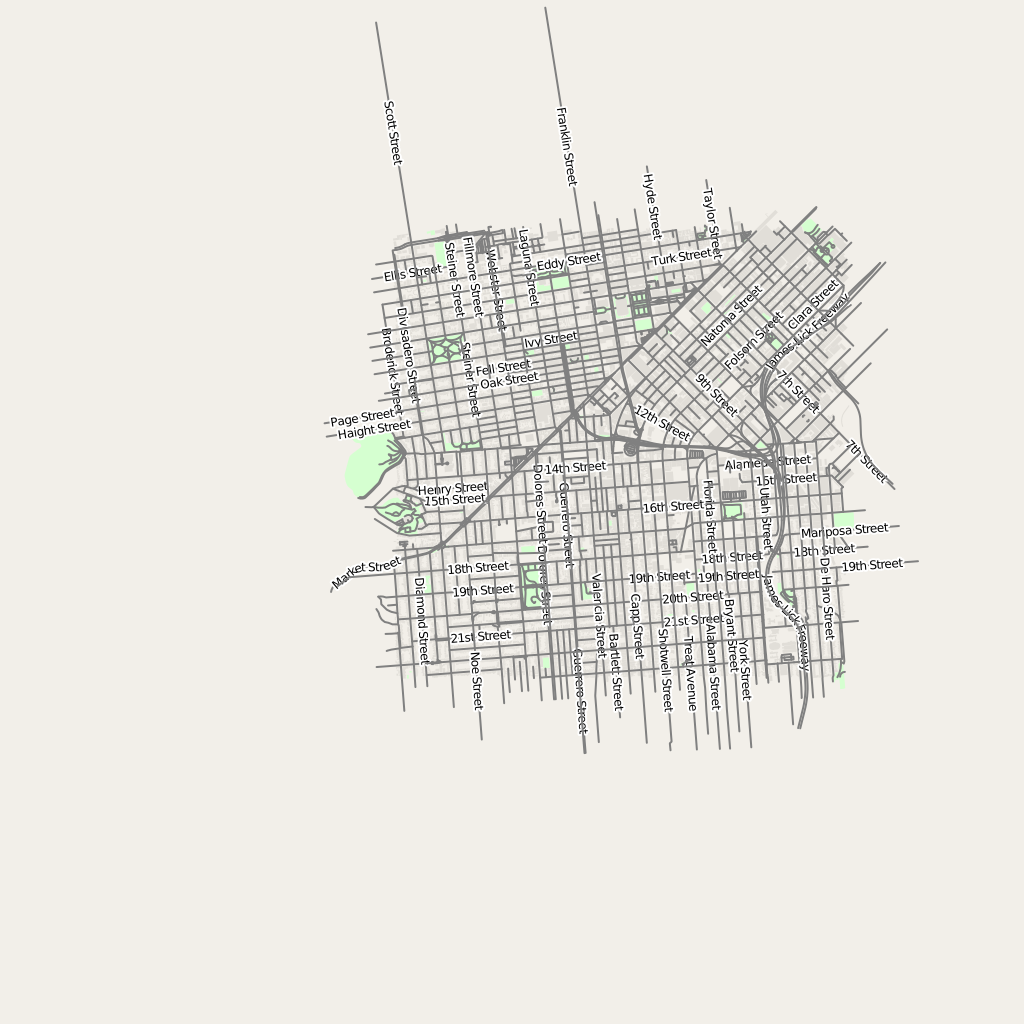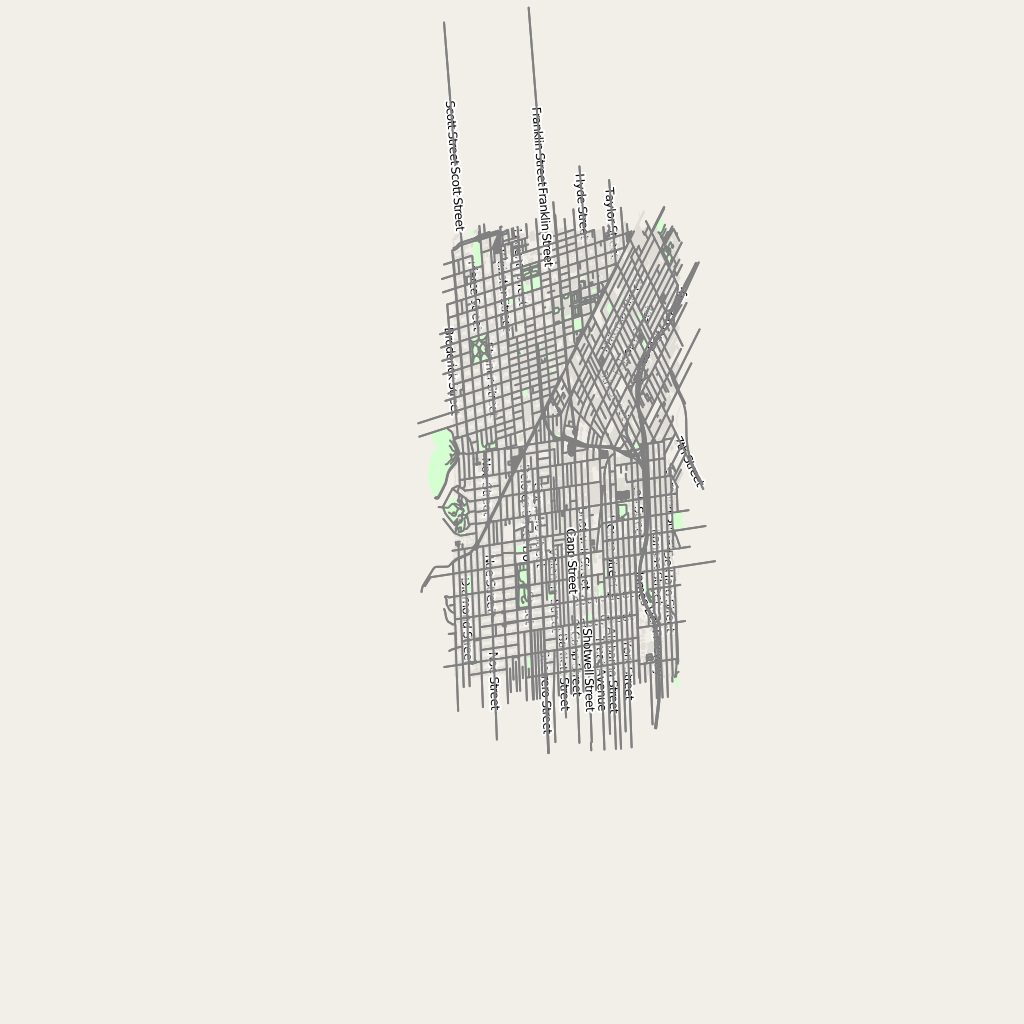# Beginning to make a custom map projection with mapnik and proj.4

## Installing mapnik and linking to a custom, self-compiled proj.4 library

I manually compiled proj.4 from downloaded source code.

I edited the Homebrew formula for mapnik2 (couldn’t get it to work with mapnik 3) so that it would link the version of proj.4 I just compiled by setting the location of these libraries to where proj.4 `make install` puts them and not where Homebrew expects them:

``````"PROJ_INCLUDES=/usr/local/proj/include",
"PROJ_LIBS=/usr/local/proj/lib",
``````

Then I compiled and installed mapnik, using the cairo option because that seems to be the only way to get Homebrew to compile it/relink the proj.4 plibraries. Since the python driver for mapnik was always looking for the libraries in the same location, this will just work – but I need to force Python to reload them, which seems to require restarting the Python kernel.

Finally, if you try to load OSM data with this custom-built mapnik, you’ll get an error like the following:

``````RuntimeError: Could not create datasource for type: 'osm'  encountered during parsing of layer 'building' in Layer at line 40 of 'mapnik_style.xml'
``````

This can be fixed by adding `"INPUT_PLUGINS=osm"` to the list of arguments used by `scons`.

### The final mapnik2.rb formula

``````class Mapnik2 < Formula
desc "Toolkit for developing mapping applications"
homepage "http://www.mapnik.org/"
url "https://s3.amazonaws.com/mapnik/dist/v2.2.0/mapnik-v2.2.0.tar.bz2"
revision 2

bottle do
sha256 "b555f843463c44234c82571c15cd9cc8345a8c30cea415cccbaccfd273beae55" => :el_capitan
sha256 "0b98b9139c184c9d58cab3fd510df54a17ca722e15897ba20dabecd13a3c641a" => :yosemite
end

# compile error in bindings/python/mapnik_text_placement.cpp
# https://github.com/mapnik/mapnik/issues/1973
patch :DATA

# boost 1.56 compatibility
# concatenated from https://github.com/mapnik/mapnik/issues/2428
patch do
sha256 "40e83052ae892aa0b134c09d8610ebd891619895bb5f3e5d937d0c48ed42d1a6"
end

depends_on "pkg-config" => :build
depends_on "freetype"
depends_on "libpng"
depends_on "libtiff"
depends_on "icu4c"
depends_on "jpeg"
depends_on "boost159"
depends_on "boost-python159"
depends_on "gdal" => :optional
depends_on "postgresql" => :optional
depends_on "cairo" => :optional
depends_on "py2cairo" if build.with? "cairo"

conflicts_with "mapnik", :because => "Differing versions of the same formula"

def install
icu = Formula["icu4c"].opt_prefix
boost = Formula["boost159"].opt_prefix
proj = Formula["proj"].opt_prefix
jpeg = Formula["jpeg"].opt_prefix
libpng = Formula["libpng"].opt_prefix
libtiff = Formula["libtiff"].opt_prefix
freetype = Formula["freetype"].opt_prefix

# mapnik compiles can take ~1.5 GB per job for some .cpp files
# so lets be cautious by limiting to CPUS/2
jobs = ENV.make_jobs.to_i
jobs /= 2 if jobs > 2

args = ["CC=\"#{ENV.cc}\"",
"CXX=\"#{ENV.cxx}\"",
"JOBS=#{jobs}",
"PREFIX=#{prefix}",
"ICU_INCLUDES=#{icu}/include",
"ICU_LIBS=#{icu}/lib",
"PYTHON_PREFIX=#{prefix}", # Install to Homebrew's site-packages
"JPEG_INCLUDES=#{jpeg}/include",
"JPEG_LIBS=#{jpeg}/lib",
"PNG_INCLUDES=#{libpng}/include",
"PNG_LIBS=#{libpng}/lib",
"TIFF_INCLUDES=#{libtiff}/include",
"TIFF_LIBS=#{libtiff}/lib",
"BOOST_INCLUDES=#{boost}/include",
"BOOST_LIBS=#{boost}/lib",
"PROJ_INCLUDES=/usr/local/proj/include",
"PROJ_LIBS=/usr/local/proj/lib",
"FREETYPE_CONFIG=#{freetype}/bin/freetype-config",
"INPUT_PLUGINS=osm"
]

if build.with? "cairo"
args << "CAIRO=True" # cairo paths will come from pkg-config
else
args << "CAIRO=False"
end
args << "GDAL_CONFIG=#{Formula["gdal"].opt_bin}/gdal-config" if build.with? "gdal"
args << "PG_CONFIG=#{Formula["postgresql"].opt_bin}/pg_config" if build.with? "postgresql"

# system "python", "scons/scons.py", "INPUT_PLUGINS=all"
system "python", "scons/scons.py", "configure", *args
system "python", "scons/scons.py", "install"
end

test do
system bin/"mapnik-config", "-v"
end
end

__END__
diff --git a/bindings/python/mapnik_text_placement.cpp b/bindings/python/mapnik_text_placement.cpp
index 0520132..4897c28 100644
--- a/bindings/python/mapnik_text_placement.cpp
+++ b/bindings/python/mapnik_text_placement.cpp
@@ -194,7 +194,11 @@ struct ListNodeWrap: formatting::list_node, wrapper<formatting::list_node>
ListNodeWrap(object l) : formatting::list_node(), wrapper<formatting::list_node>()
{
stl_input_iterator<formatting::node_ptr> begin(l), end;
-        children_.insert(children_.end(), begin, end);
+        while (begin != end)
+        {
+            children_.push_back(*begin);
+            ++begin;
+        }
}

/* TODO: Add constructor taking variable number of arguments.
``````

## Writing a custom map projection

Proj.4 has many source files, prefixed with PJ_ that are used to convert coordinates from spherical or ellipsoidal points into rectangular ones. As an example, below is the source code to the Mercator implementation, PJ_merc.c:

``````#define PJ_LIB__
#include	<projects.h>
PROJ_HEAD(merc, "Mercator") "\n\tCyl, Sph&Ell\n\tlat_ts=";
#define EPS10 1.e-10
FORWARD(e_forward); /* ellipsoid */
if (fabs(fabs(lp.phi) - HALFPI) <= EPS10) F_ERROR;
xy.x = P->k0 * lp.lam;
xy.y = - P->k0 * log(pj_tsfn(lp.phi, sin(lp.phi), P->e));
return (xy);
}
FORWARD(s_forward); /* spheroid */
if (fabs(fabs(lp.phi) - HALFPI) <= EPS10) F_ERROR;
xy.x = P->k0 * lp.lam;
xy.y = P->k0 * log(tan(FORTPI + .5 * lp.phi));
return (xy);
}
INVERSE(e_inverse); /* ellipsoid */
if ((lp.phi = pj_phi2(P->ctx, exp(- xy.y / P->k0), P->e)) == HUGE_VAL) I_ERROR;
lp.lam = xy.x / P->k0;
return (lp);
}
INVERSE(s_inverse); /* spheroid */
lp.phi = HALFPI - 2. * atan(exp(-xy.y / P->k0));
lp.lam = xy.x / P->k0;
return (lp);
}
FREEUP; if (P) pj_dalloc(P); }
ENTRY0(merc)
double phits=0.0;
int is_phits;

if( (is_phits = pj_param(P->ctx, P->params, "tlat_ts").i) ) {
phits = fabs(pj_param(P->ctx, P->params, "rlat_ts").f);
if (phits >= HALFPI) E_ERROR(-24);
}
if (P->es) { /* ellipsoid */
if (is_phits)
P->k0 = pj_msfn(sin(phits), cos(phits), P->es);
P->inv = e_inverse;
P->fwd = e_forward;
} else { /* sphere */
if (is_phits)
P->k0 = cos(phits);
P->inv = s_inverse;
P->fwd = s_forward;
}
ENDENTRY(P)
``````

Note the use of function definition compiler macros. Also note that the coordinates returned (`(xy)`) are somewhat arbitrary – they seem to vary dramatically from projection to projection.

Let’s try dividing the output x coordinate by two. One condition that proj.4/mapnik does enforce is a 1:1 aspect ratio between x and y, so this should compress the output image. Note that we had to multiply it by two in the inverse functions as well.

``````#define PJ_LIB__
#include	<projects.h>
PROJ_HEAD(merc, "Mercator") "\n\tCyl, Sph&Ell\n\tlat_ts=";
#define EPS10 1.e-10
FORWARD(e_forward); /* ellipsoid */
if (fabs(fabs(lp.phi) - HALFPI) <= EPS10) F_ERROR;
xy.x = P->k0 * lp.lam / 2;
xy.y = - P->k0 * log(pj_tsfn(lp.phi, sin(lp.phi), P->e));
return (xy);
}
FORWARD(s_forward); /* spheroid */
if (fabs(fabs(lp.phi) - HALFPI) <= EPS10) F_ERROR;
xy.x = P->k0 * lp.lam / 2;
xy.y = P->k0 * log(tan(FORTPI + .5 * lp.phi));
return (xy);
}
INVERSE(e_inverse); /* ellipsoid */
if ((lp.phi = pj_phi2(P->ctx, exp(- xy.y / P->k0), P->e)) == HUGE_VAL) I_ERROR;
lp.lam = 2 * xy.x / P->k0;
return (lp);
}
INVERSE(s_inverse); /* spheroid */
lp.phi = HALFPI - 2. * atan(exp(-xy.y / P->k0));
lp.lam = 2 * xy.x / P->k0;
return (lp);
}
FREEUP; if (P) pj_dalloc(P); }
ENTRY0(merc)
double phits=0.0;
int is_phits;

if( (is_phits = pj_param(P->ctx, P->params, "tlat_ts").i) ) {
phits = fabs(pj_param(P->ctx, P->params, "rlat_ts").f);
if (phits >= HALFPI) E_ERROR(-24);
}
if (P->es) { /* ellipsoid */
if (is_phits)
P->k0 = pj_msfn(sin(phits), cos(phits), P->es);
P->inv = e_inverse;
P->fwd = e_forward;
} else { /* sphere */
if (is_phits)
P->k0 = cos(phits);
P->inv = s_inverse;
P->fwd = s_forward;
}
ENDENTRY(P)
``````

## Compiling

First, in the proj.4 directory, run

``````make
make install
``````

Then rebuild mapnik to relink the proj.4 (maybe there’s a better way of doing this?):

``````brew uninstall homebrew/versions/mapnik2
brew install homebrew/versions/mapnik2 --with-cairo
``````

## Using the new map projection

In your mapnik XML stylesheet, set the projection like so (this corresponds to web mercator):

``````<Map background-color="#f2efe9" srs="+proj=merc +a=6378137 +b=6378137 +lat_ts=0.0 +lon_0=0.0 +x_0=0.0 +y_0=0 +k=1.0 +units=m +nadgrids=@null +wktext +no_defs">
``````

With the original PJ_merc.c:With the modified/stretched PJ_merc.c:Obviously this is only the tip of the iceberg for defining a map projection – you just need a set of functions for performing the forward and inverse projection, and you’re ready to start rendering real maps with real data. You can even render tiled maps for use with maps browser such as Leaflet.